## Overview

Overview

Presentation of Data  25

3Presentation of Data

3.1Introduction

3.2Classification

3.3Tabulation

3.4Frequency Distribution

3.5Cumulative Frequency

3.6​​  Diagram and Graphs​​

(a)​​ Bar diagram​​

(b)​​ Pie Diagram​​

(c)​​ Histogram​​

(d)​​ Frequency Curve or Frequency Polygon​​

(e)​​ Ogives

Review Question

In previous we learnt about primary data, secondary data, tools of collecting primary data and various sources of secondary data. Once the data has been collected the logical next step is to present it in a form that looks attractive and helps the researcher analyze it with ease. The commonly used forms of presentation of the data are:​​

1.Classification,​​

2.Tabulation,​​

3.Frequency Distribution,​​

4.Cumulative Frequency Distribution,​​

5.Diagrams and graphs.

This form of presentation is used when a large body of numerical data is given in textual form or data arrays. The data is divided into smaller groups or classes on the basis of size or degree or some common characteristic of the variant.

Classification is invariably accompanied by tabulation and involves a process called frequency distribution.The number of times a certain value of the variant occurs in the raw data is called its frequency.

For example, in the raw data 8, 7, 5, 8, 4, 8, 3, 10, 8, the value 8 occurs 4 times. So frequency of 8 is 4.

The process of placing classified data into tabular form is known as tabulation. A table is a symmetric arrangement of statistical data in rows and columns. Rows are horizontal arrangements whereas columns are vertical arrangements. It may be simple, double or complex depending upon the type of classification.

Types of Tabulation:

The types of tabulation​​ are explained as follows:

(1)Simple Tabulation or One-way Tabulation:​​ When the data are tabulated to one characteristic, it is said to be a simple tabulation or one-way tabulation.​​ For example: Tabulation of data on the population of the world classified by one characteristic like religion is an example of a simple tabulation.

(2)Double Tabulation or Two-way Tabulation:​​ When the data are tabulated according to two characteristics at a time, it is said to be a double tabulation or two-way tabulation.​​ For example: Tabulation of data on the population of the world classified by two characteristics like religion and sex is an example of a double tabulation.

(3)Complex Tabulation:​​ When the data are tabulated according to many characteristics, it is said to be a complex tabulation.​​ For example: Tabulation of data on the population of the world classified by three or more characteristics like religion, sex and literacy, etc. is an example of a complex tabulation.

A statistical data may consists of a list of numbers related to a research. Among those numbers, few may be repeated twice and even more than twice. The repetition of number is a data set is termed as frequency of that particular number or the variable in which that number is assigned. The frequencies of variables in a data are to be listed in a table. This table is known as frequency distribution table and the list is referred as frequency distribution.

A frequency distribution is an orderly arrangement of data classified according to the magnitude of the observations. When the data are grouped into classes of appropriate size indicating the number of observations in each class we get a frequency distribution. By forming frequency distribution, we can summarize the data effectively. It is a method of presenting the data in a summarized form. Frequency distribution is also known as Frequency table. Frequency distribution helps us:​​

​​ To analyze the data.

​​ To estimate the frequencies of the population on the basis of the ample.

​​ To facilitate the computation of various statistical measures.

Frequency distribution is divided into two:​​ Grouped and Ungrouped

(1)Grouped:​​ Grouped frequency is frequency where class interval are formed. The values of class interval is called Class Limits. The minimum value is lower class limit and maximum value is upper class limit.​​ Grouped frequency is​​ again​​ divided into two:

(a)Inclusive Method:​​ In this, the class intervals are not in continuous formed. It is known as Discrete Classes.

(b)Exclusive Method:​​ In this, the class intervals are in continuous formed.​​ It is known as Continuous Classes.

(2)Ungrouped:​​ Ungrouped Frequency is frequency where no class interval is formed.​​ Thus,​​ there is no lower and upper limits.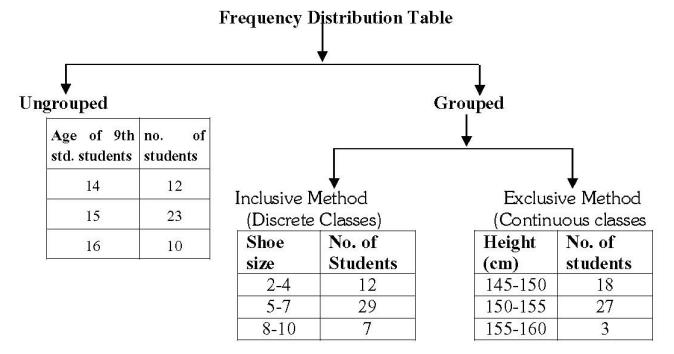Solved Examples

Ex.(1)In a ‘tree plantation’ project of a certain school there are 45 students of 'Harit​​ Sena.' The record of trees planted​​ by each student is given below:​​

3, 5, 7, 6, 4, 3, 5, 4, 3, 5, 4, 7, 5 , 3, 6, 6, 5, 3, 4, 5, 7, 3, 5, 6, 4, 4, 3, 5, 6, 6, 4, 3, 5, 7, 3, 4, 5, 7, 6, 4, 3, 5, 4, 4, 7.​​

Prepare​​ an ungrouped​​ frequency distribution table of the data.​​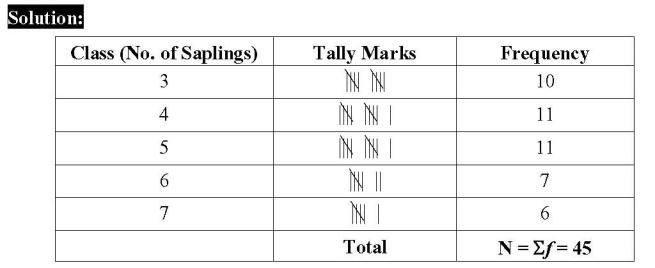Ex.​​  (2)​​  The value of​​ up​​ to 50 decimal places is given below:​​

3.14159265358979323846264338327950288419716939937510​​

From this information prepare an ungrouped frequency distribution table of digits appearing after the decimal point.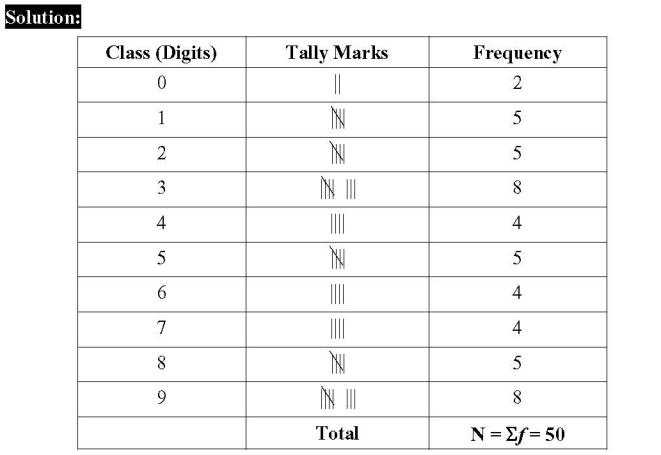Ex.(3)​​  In a college, 46​​ students of 12thstandard, were told to measure the lengths of the pen​​ in their compass-boxes in centimetres. The data collected was as follows.​​

16, 15, 7, 4.5, 8.5, 5.5, 5, 6.5, 6, 10, 12, 13, 4.5, 4.9, 16, 11, 9.2, 7.3, 11.4, 12.7, 13.9, 16, 5.5, 9.9, 8.4, 11.4, 13.1, 15, 4.8, 10, 7.5, 8.5, 6.5, 7.2, 4.5, 5.7, 16, 5.7, 6.9, 8.9, 9.2, 10.2, 12.3, 13.7, 14.5, 10​​

By taking inclusive classes 0-5, 5-10, 10-15.... Prepare a grouped frequency distribution table.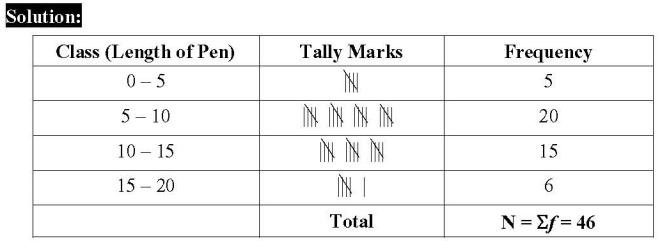Ex.​​  (4)​​  In the tables given below, class-mark and frequencies is given. Construct the frequency tables taking​​ inclusive and exclusive classes:​​

(1) ​​​​  ​​ ​​ ​​​​

 Class Width(x)​​ Frequency(f)​​ 5 9 15 9 25 15 35 13

Solution:

Class​​ Width​​  =​​  The difference between any two consecutive class.

​​ ​​ ​​ ​​ ​​ ​​ ​​ ​​ ​​ ​​ ​​ ​​ ​​ ​​ ​​ ​​ ​​ ​​ ​​ ​​ ​​​​   =​​  15 – 5

​​ ​​ ​​ ​​ ​​ ​​ ​​ ​​ ​​ ​​ ​​ ​​ ​​ ​​ ​​ ​​ ​​ ​​ ​​ ​​ ​​ ​​​​   =​​  10

​​  Class Width​​  =​​  10

​​  Divide​​  = ​​ 102​​ = 5

Now, Subtract 5 to each class and add 5 to each class to get class interval.

Frequency Distribution Table,

​​ By Exclusive Method:

 Class Interval Class Marks(x) Frequency(f) 0 – 10 5 9 10 – 20 15 9 20 – 30 25 15 30 – 40 35 13

​​ By Inclusive Method:

 Class Interval Class Marks(x) Frequency(f) 0.5 – 9.5 5 9 10.5 – 19.5 15 9 20.5 – 29.5 25 15 30.5 – 39.5 35 13

(2)

 Class Width​​ (x)​​ Frequency(f)​​ 22 6 24 7 26 13 28 4

Solution:

Class Width​​  =​​  The difference between any two consecutive class.

​​ ​​ ​​ ​​ ​​ ​​ ​​ ​​ ​​ ​​ ​​ ​​ ​​ ​​ ​​ ​​ ​​ ​​ ​​ ​​ ​​​​   =​​  24 – 22​​

​​ ​​ ​​ ​​ ​​ ​​ ​​ ​​ ​​ ​​ ​​ ​​ ​​ ​​ ​​ ​​ ​​ ​​ ​​ ​​ ​​​​   =​​  2

​​  Class Width=2

​​  Divide​​  = ​​ 22​​ = 1

Now, Subtract 1 to each class and add 1 to each class to get class interval.

Frequency Distribution Table,

​​ By Exclusive Method:

 Class Interval Class Marks(x) Frequency(f) 21 – 23 22 6 23 – 25 24 7 25 – 27 26 13 27 - 29 28 4

​​ By Inclusive Method:

 Class Interval Class Marks(x) Frequency(f) 21.5 – 22.5 22 6 23.5 – 24.5 24 7 25.5 – 26.5 26 13 27.5 – 28.5 28 4

Cumulative frequency is the running total of the​​ frequencies. Cumulative Frequencies are number of observations above or below of a certain value.​​

There are two types of Cumulative Frequency.

(1)Cumulative Frequency – Less than type:​​ Number of observation less than the upper boundary of a class is called “Less than type” cumulative frequency of that class.​​

(2)Cumulative Frequency – More than type:​​ Number of observation more than or equal to the lower boundary of a class is called “More than type” cumulative frequency of that class.​​

Solved Examples:

Ex.​​  (1)​​  Calculate less than type cumulative frequency:

 Class Interval Frequency 0 – 10 3 10 – 20 10 20 – 30 7 30 – 40 8 40 – 50 2

Solution:

 Class Interval Frequency Cumulative Frequency Less than type 0 – 10 3 3 10 – 20 10 3 + 10 = 13 20 – 30 7 13 + 7 = 20 30 – 40 8 20 + 8 = 28 40 – 50 2 28 + 2 =​​ 30 N = 30

Ex.​​  (2)​​  Calculate​​ more​​ than type cumulative frequency:

 Class Interval​​ Frequency 0 – 10 3 10 – 20 10 20 – 30 7 30 – 40 8 40 – 50 2

Solution:

 Class Interval Frequency Cumulative Frequency More​​ than type 0 – 10 3 27 + 3 =​​ 30 10 – 20 10 17 + 10 = 27 20 – 30 7 10 + 7 = 17 30 – 40 8 2 + 8 = 10 40 – 50 2 2 N = 30

Ex.​​  (3)Calculate Less than and More than type cumulative frequency:

 Class Interval Frequency 150 – 153 5 153 – 156 7 156 – 159 15 159 – 162 10 162 – 165 5 165 – 168 3

Solution:

 Class Interval Frequency Cumulative Frequency Less than type Cumulative Frequency More than type 150 – 153 5 5 40 + 5 =​​ 45 153 – 156 7 5 + 7 = 12 33 + 7 = 40 156 – 159 15 12 + 15 = 27 18 + 15 = 33 159 – 162 10 27 + 10 = 37 8 + 10 = 18 162 – 165 5 37 + 5 = 42 3 + 5 = 8 165 – 168 3 42 + 3 =​​ 45 3 N = 45

Ex.​​  (4)​​  Calculate Less than and More than type cumulative frequency:

 Class Interval Frequency 1000 – 5000 45 5000 – 10000 19 10000 – 15000 16 15000 – 20000 2 20000 – 25000 5

Solution:

 Class Interval Frequency Cumulative Frequency Less than type Cumulative Frequency More than type 1000 – 5000 45 45 42 + 45 = 87 5000 – 10000 19 45 + 19 = 64 23 + 19 = 42 10000 – 15000 16 64 + 16 = 80 7 + 16 = 23 15000 – 20000 2 80 + 2 = 82 5 + 2 = 7 20000 – 25000 5 82 + 5 = 87 5 N = 87

Diagrams and graphs are geometrical representations of a data. They do not involve any complex calculations and so are easy to draw. They are pleasing to the eye, give a bird’s – eye view of the whole data and highlight all its salient features. They are easy to interpret and facilitate comparisons between different sets of data. Some of the commonly used diagrams and graphs are:

(a)Bar Diagrams

(b)Pie Diagram (or Pie chart)

(c)Histogram

(d)Frequency Curve​​ or​​ Frequency Polygon​​

(e)Ogives (cumulative frequency curve)

A bar graph (also known as a bar chart or bar diagram) is a visual tool that uses bars to compare data among categories. A bar graph may run horizontally or vertically. The important thing to know is that the longer the bar, the greater its value.

Bar graphs consist of two axes. On a vertical bar graph, as shown above, the horizontal axis (or x-axis) shows the data categories. In this example, they are years. The vertical axis (or y-axis) is the scale. The coloured bars are the data series.Bar diagrams are of many types such as:​​

(1)Simple bar diagram:​​ A simple bar chart is used to represent data involving only one variable classified on a spatial, quantitative or temporal basis. In a simple bar chart, we make bars of equal width but variable length, i.e. the magnitude of a quantity is represented by the height or length of the bars.

(2)Multiple bar diagram:​​ In a multiple bars diagram two or more sets of inter-related data are represented (multiple bar diagram facilitates comparison between more than one phenomena). The technique of making a simple bar chart is used to draw this diagram but the difference is that we use different shades, colours, or dots to distinguish between different phenomena.

(3)Subdivided bar diagram:​​ A sub-divided or component bar chart is used to represent data in which the total magnitude is divided into different or components. In this diagram, first we make simple bars for each class taking the total magnitude in that class and then divide these simple bars into parts in the ratio of various components.

(4)Percentage bar diagram:​​ In drawing a percentage bar chart, bars of length equal to 100 for each class are drawn in the first step and sub-divided into the proportion of the percentage of their component in the second step. The diagram so obtained is called a percentage component bar chart or percentage stacked bar chart. This type of chart is useful to make comparisons in components holding the difference of total constants.

Solved Examples:

Ex. (1)​​ With the help of given statistical data prepare a simple bar graph:

 Years 1971 1981 1991 2001 2011 Population (in crores) 55 68 85 103 121

Solution: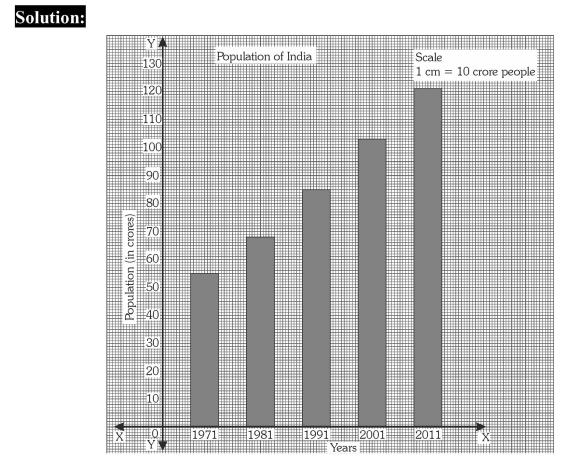Ex. (2) Draw a simple bar graph by to represent the following data:​​

 Years 2000 – 2001 2001 - 2002 2002 – 2003 2003 – 2004 2004 – 2005 Per Capita Income (in Rs.) 18000 19000 20000 24000 27000

Solution:

1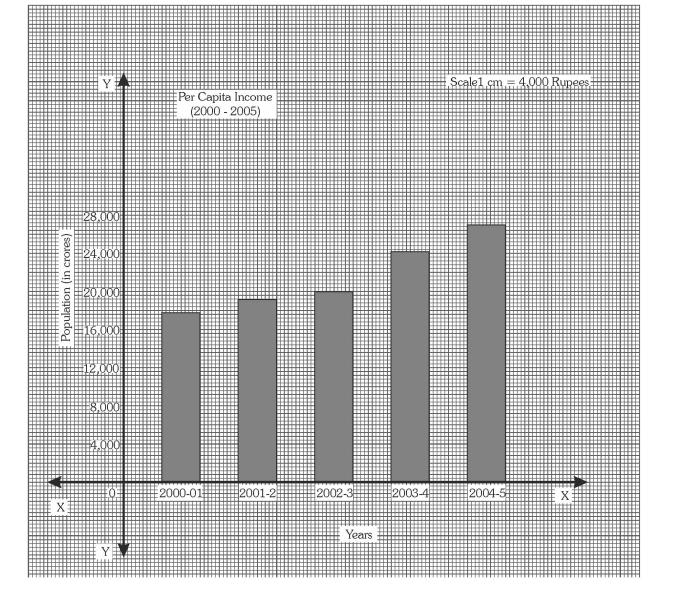Ex. (3)​​ Following data gives imports and exports of India​​ for​​ a few years. Construct a suitable diagram to represent the given data:

(BMS, April, 2017)​​

 Year Rupees in crores Imports Exports 1994 – 1995 17,170 11,850 1995 – 1996 19,760 11,010 1996 – 1997 20,080 12,550 1997 – 1998 22,330 15,710

Solution: